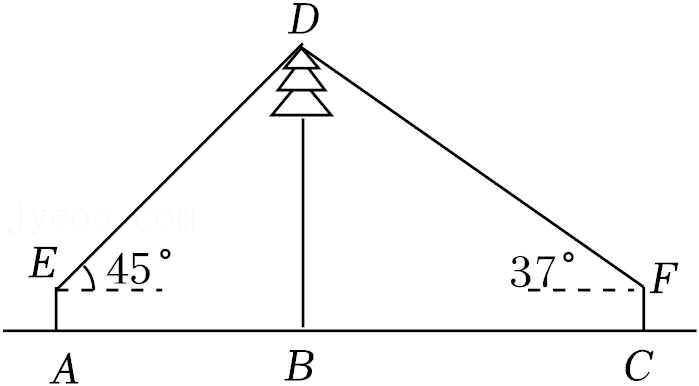【答案】 解: 连接 $\mathrm{EF}$, 交 $\mathrm{BD}$ 于点 $\mathrm{M}$, 则 $\mathrm{EF} \perp \mathrm{BD}, \mathrm{AE}=\mathrm{BM}=$ $\mathrm{CF}=1.6$ 米,

$\therefore \mathrm{EM}=\mathrm{DM}$,

$$\therefore \mathrm{DB}=12+1.6=13.6 \text { (米), }$$